# Energy Conservation

Consider a small-amplitude transverse wave propagating along a uniform string of infinite length, tension, and mass per unit length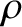. (See Section 4.3.) Let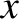measure distance along the string, and let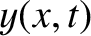be the transverse wave displacement. As we have seen,satisfies the wave equation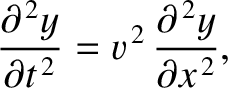(6.23)

where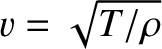is the phase velocity of traveling waves on the string.

Consider a section of the string lying between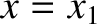and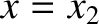. The kinetic energy of this section is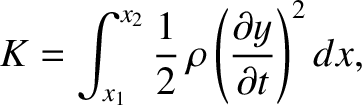(6.24)

because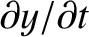is the string's transverse velocity (and the longitudinal velocity is assumed to be negligibly small). The potential energy is the work done in stretching the section, which is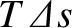, where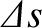is the difference between the section's stretched and unstretched lengths. Here, it is assumed that the tension remains approximately constant as the section is stretched. An element of length of the string is written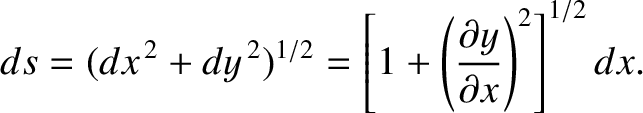(6.25)

Hence,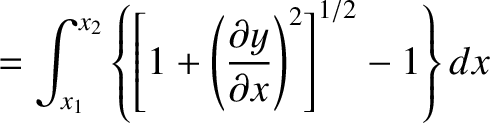(6.26)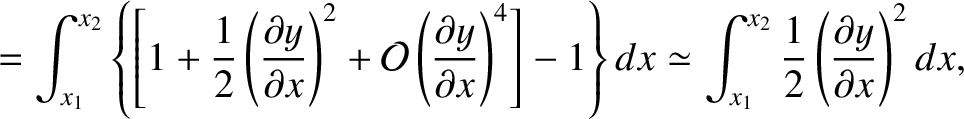(6.27)

because it is assumed that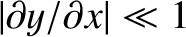(i.e., the transverse displacement is sufficiently small that the string remains almost parallel to the-axis). Thus, the potential energy of the section is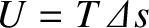, or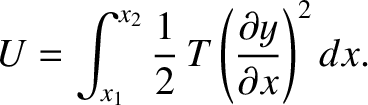(6.28)

It follows that the total energy of the section is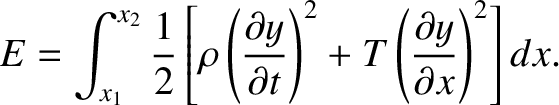(6.29)

Multiplying the wave equation, (6.23), by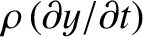, we obtain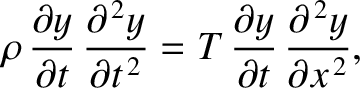(6.30)

because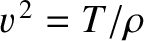. This expression yields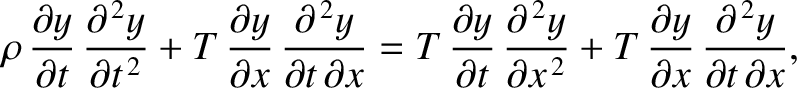(6.31)

which can be written in the form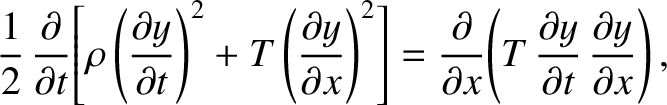(6.32)

or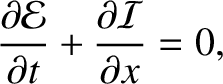(6.33)

where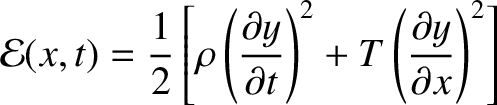(6.34)

is the energy density (i.e., the energy per unit length) of the string, and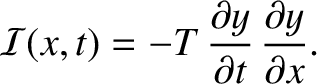(6.35)

Finally, integrating Equation (6.33) infrom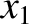to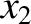, we obtain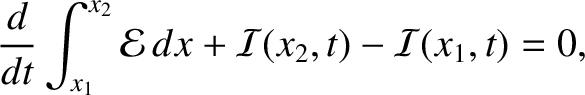(6.36)

or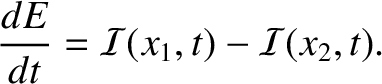(6.37)

Here,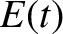is the energy stored in the section of the string lying betweenand. [See Equation (6.29).] If we interpret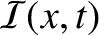as the instantaneous energy flux (i.e., rate of energy flow) in the positive-direction, at positionand time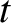, then the previous equation can be recognized as a declaration of energy conservation. Basically, the equation states that the rate of increase in the energy stored in the section of the string lying betweenand, which is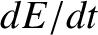, is equal to the difference between the rate at which energy flows into the left end of the section, which is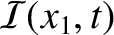, and the rate at which it flows out of the right end, which is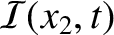. Incidentally, the string conserves energy because it lacks any mechanism for energy dissipation. The same is true of the other wave media discussed in this chapter.

Consider a wave propagating in the positive-direction of the form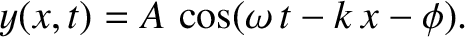(6.38)

According to Equation (6.35), the energy flux associated with this wave is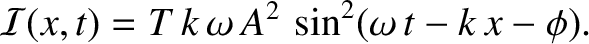(6.39)

Thus, the mean energy flux is written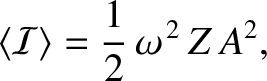(6.40)

where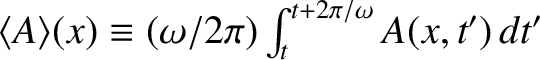represents an average over a period of the wave oscillation. Here, use has been made of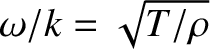, as well as the result that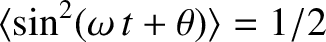for all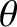. Moreover, the quantity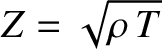(6.41)

is known as the characteristic impedance of the string. The units ofare force over velocity. Thus, the string impedance measures the typical tension required to produce a unit transverse velocity. Finally, according to Equation (6.40), a traveling wave propagating in the positive-direction is associated with a positive energy flux. In other words, the wave transports energy in the positive-direction.

Consider a wave propagating in the negative-direction of the general form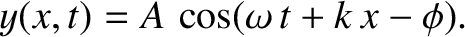(6.42)

It can be demonstrated, from Equation (6.35), that the mean energy flux associated with this wave is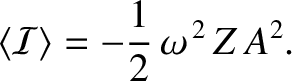(6.43)

The fact that the energy flux is negative means that the wave transports energy in the negative-direction.

Suppose that we have a superposition of a wave of amplitude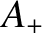propagating in the positive-direction, and a wave of amplitude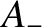propagating in the negative-direction, so that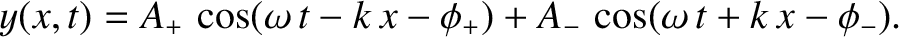(6.44)

According to Equation (6.35), the instantaneous energy flux is written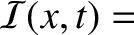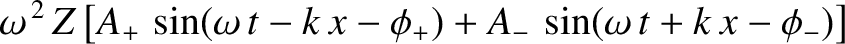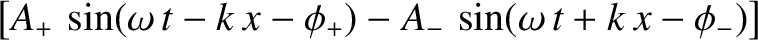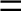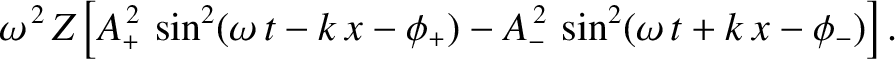(6.45)

Hence, the mean energy flux,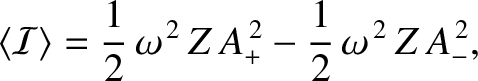(6.46)

is the difference between the independently calculated mean fluxes associated with the waves traveling to the right (i.e., in the positive-direction) and to the left. Recall, from the previous section, that a standing wave is a superposition of two traveling waves, of equal amplitude and frequency, propagating in opposite directions. It immediately follows, from the previous expression, that a standing wave has zero associated net energy flux. In other words, a standing wave does not give rise to net energy transport.

We saw earlier, in Section 5.3, that a small-amplitude longitudinal wave in a thin elastic rod satisfies the wave equation,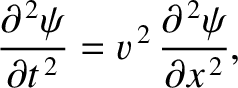(6.47)

where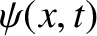is the longitudinal wave displacement,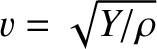the phase velocity of traveling waves along the rod,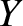the Young's modulus, andthe mass density. Using similar analysis to that just employed, we can derive an energy conservation equation of the form (6.33) from this wave equation, where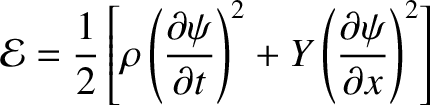(6.48)

is the wave energy density (i.e., the energy per unit volume), and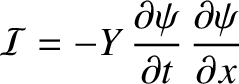(6.49)

the wave energy flux (i.e., the rate of energy flow per unit area) in the positive-direction. For a traveling wave of the form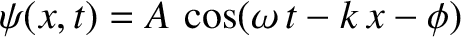, the previous expression yields(6.50)

where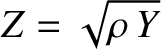(6.51)

is the impedance of the rod. The units ofare pressure over velocity. Hence, in this case, the impedance measures the typical pressure in the rod required to produce a unit longitudinal velocity. Analogous arguments reveal that the impedance of an ideal gas of density, pressure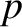, and ratio of specific heats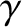, is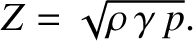(6.52)

(See Section 5.4.)